### Home > CALC > Chapter Ch7 > Lesson 7.4.1 > Problem7-176

7-176.
1. Etube is tending to the orange trees on his lot. Unfortunately, the trees are becoming infected and dying. The rate at which the trees are being infected per month is given by 3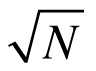where N is the number of living trees. Homework Help ✎

1. If at t = 0 there are 3,000 living trees, set up the appropriate differential equation to represent the number of living trees N as a function of t, where t is measured in months.

2. Solve the initial value problem to find the number of living trees after t months.

3. How many trees will be infected at t = 5 months?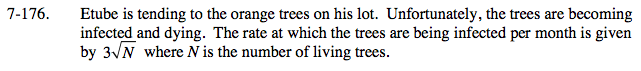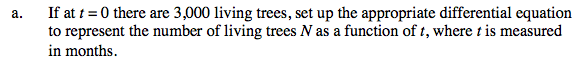The initial condition is not relevant, yet.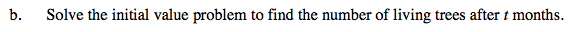Separate the variables so that all of the 'N' variables are on one side of the equation and all of the 't' variables are on the other.
Then integrate both sides of the equation and solve for 'N'. Use the initial condition to solve for C.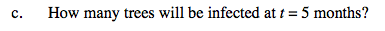Evaluate.